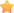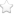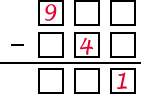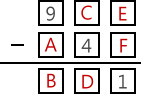×927-346=581
927-546=381→C+10-4=D
C+6=D

(3, 5, 6, 7)唯一符合的就是(3, 5)或(5, 3)兩者皆可

C=2, D=8, E=7, F=6, 而(A, B)=(3, 5)或(5, 3)

（你有更好的解法嗎？歡迎到粉絲團遊戲學校去跟大家分享喔！）